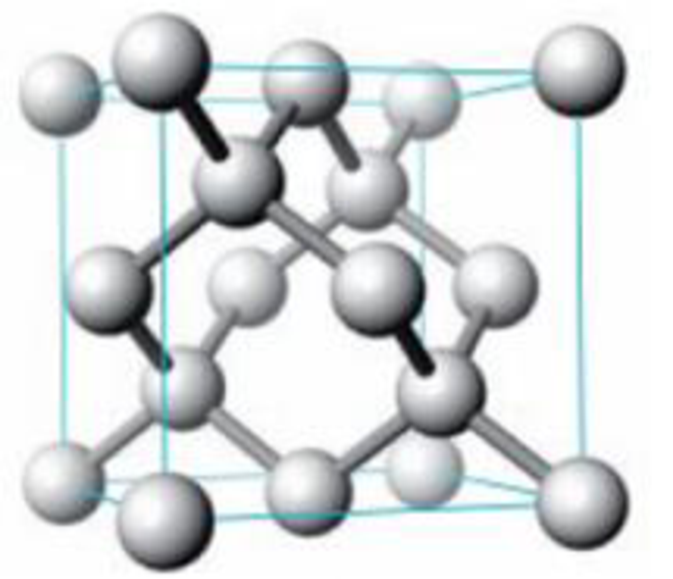Chapter 12, Problem 51GQ

Chapter
Section
Textbook Problem

The solid-state structure of silicon is shown below.Unit cell for silicon (a) Describe this crystal as pc, bcc, or fcc. (b) What type of holes are occupied in the lattice? (c) How many Si atoms are there per unit cell? (d) Calculate the density of silicon in g/cm3 (given that the cube edge has a length of 543.1 pm). (e) Estimate the radius of the silicon atom. (Note: The Si atoms on the edges do not touch one another.)

(a)

Interpretation Introduction

Interpretation:

Given silicon crystal has to be described for PC, BCC or FCC.

Concept introduction:

• An ionic radii are the radius of an atom's ion in ionic crystals structure.
• An ionic solid is made up cations and anions held together by electrostatic forces in a rigid array or lattice.
• Positive charge ions are cations and negative charge ions are anions.
• Lattice Energy is mainly depends on the charge on the ion and radius or size of the ion.
• Ionic radius increases from top to bottom on the periodic table.

Ionic radius decreases from left to right the periodic table.

• The density of the unit cell:

Massvolume = density

• The face-centered cubic system:

It has lattice points on the faces of the cube, that each gives exactly one half contributions, in addition to the corner lattice points, giving a total of 4 lattice points per unit cell

Explanation

The types of the lattice is face center cubic crystal, because It has lattice points on the faces of the cube, that each giv...

(b)

Interpretation Introduction

Interpretation:

The types of holes that are occupied in the lattice has to be determined.

Concept introduction:

• An ionic radii are the radius of an atom's ion in ionic crystals structure.
• An ionic solid is made up cations and anions held together by electrostatic forces in a rigid array or lattice.
• Positive charge ions are cations and negative charge ions are anions.
• Lattice Energy is mainly depends on the charge on the ion and radius or size of the ion.
• Ionic radius increases from top to bottom on the periodic table.

Ionic radius decreases from left to right the periodic table.

• The density of the unit cell:

Massvolume = density

• The face-centered cubic system:

It has lattice points on the faces of the cube, that each gives exactly one half contribution, in addition to the corner lattice points, giving a total of 4 lattice points per unit cell

(c)

Interpretation Introduction

Interpretation:

Number of silicon atoms per unit cell has to be determined.

Concept introduction:

• An ionic radii are the radius of an atom's ion in ionic crystals structure.
• An ionic solid is made up cations and anions held together by electrostatic forces in a rigid array or lattice.
• Positive charge ions are cations and negative charge ions are anions.
• Lattice Energy is mainly depends on the charge on the ion and radius or size of the ion.
• Ionic radius increases from top to bottom on the periodic table.

Ionic radius decreases from left to right the periodic table.

• The density of the unit cell:

Massvolume = density

• The face-centered cubic system:

It has lattice points on the faces of the cube, that each gives exactly one half contribution, in addition to the corner lattice points, giving a total of 4 lattice points per unit cell

(d)

Interpretation Introduction

Interpretation:

The density of the silicon atom has to be identified.

Concept introduction:

• An ionic radii are the radius of an atom's ion in ionic crystals structure.
• An ionic solid is made up cations and anions held together by electrostatic forces in a rigid array or lattice.
• Positive charge ions are cations and negative charge ions are anions.
• Lattice Energy is mainly depends on the charge on the ion and radius or size of the ion.
• Ionic radius increases from top to bottom on the periodic table.

Ionic radius decreases from left to right the periodic table.

• The density of the unit cell:

Massvolume = density

• The face-centered cubic system:

It has lattice points on the faces of the cube, that each gives exactly one half contribution, in addition to the corner lattice points, giving a total of 4 lattice points per unit cell

(e)

Interpretation Introduction

Interpretation:

The radius of the silicon atom has to be identified.

Concept introduction:

• An ionic radii are the radius of an atom's ion in ionic crystals structure.
• An ionic solid is made up cations and anions held together by electrostatic forces in a rigid array or lattice.
• Positive charge ions are cations and negative charge ions are anions.
• Lattice Energy is mainly depends on the charge on the ion and radius or size of the ion.
• Ionic radius increases from top to bottom on the periodic table.

Ionic radius decreases from left to right the periodic table.

• The density of the unit cell:

Massvolume = density

• The face-centered cubic system:

It has lattice points on the faces of the cube, that each gives exactly one half contribution, in addition to the corner lattice points, giving a total of 4 lattice points per unit cell

Still sussing out bartleby?

Check out a sample textbook solution.

See a sample solution

The Solution to Your Study Problems

Bartleby provides explanations to thousands of textbook problems written by our experts, many with advanced degrees!

Get Started

Explain why somebut not allof an organism's genes are expressed.

Biology: The Unity and Diversity of Life (MindTap Course List)

What is needed to designate a position?

An Introduction to Physical Science

Define the following terms: a. chromosome b. chromatin

Human Heredity: Principles and Issues (MindTap Course List)